Search
Search

# Quantum Programming 101: Superdense Coding Tutorial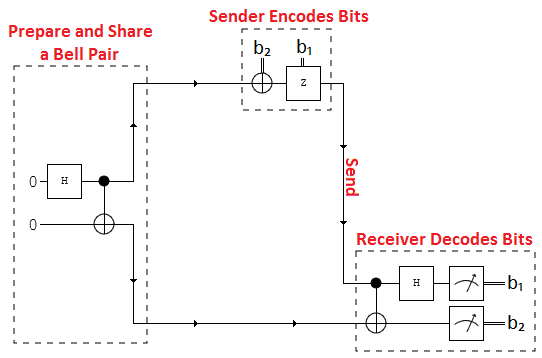# What is Superdense coding ?

Superdense coding is a quantum communications protocol that allows a user to send 2 classical bits by sending only 1 qubit.

# The protocol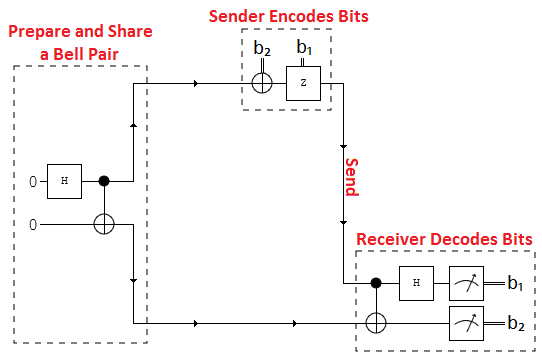# Step 1: Preparing the Bell pair

First a bell pair consisting of 2 qubits is prepared. Where q0 is the senders qubit and q1 is the receivers qubit. To do this q0 is put in to a superposition of states using a hadamard gate.

Then a CNOT operation is performed with q0 being the control and q1 being the target.

# Step 2: Encode the information on to q0

Next the sender has to encode the information they want to send on to q0 by applying certain operations to it.

• If they want to send 00 then they perform no operation.

• If they want to send 01 then they perform a Pauli-X operation where q1s state is flipped.

• If they want to send 10 then they apply a Pauli-Z gate.

• If they want to send 11 then apply a Pauli-Z gate followed by a Pauli-X gate

# Step 3: Receiver decodes the information

Next q0 is sent and the receiver has to decode the qubit. This is done by applying a CNOT where the received q0 is the control and q1 is the target. Then a hadamard gate is applied to q0.

# How to run the program

1. Copy and paste the code below in to a python file

2. Enter your API token in the IBMQ.enable_account(‘Insert API token here’) part

3. Save and run

# Code

``````print('\n Superdense Coding')
print('--------------------------\n')

from qiskit import QuantumRegister, ClassicalRegister, QuantumCircuit, execute,IBMQ

IBMQ.enable_account('INSERT TOKEN HERE')
provider = IBMQ.get_provider(hub='ibm-q')

q = QuantumRegister(2,'q')
c = ClassicalRegister(2,'c')

backend = provider.get_backend('ibmq_qasm_simulator')
print('Provider: ',backend)

#################### 00 ###########################
circuit = QuantumCircuit(q,c)

circuit.h(q) # Hadamard gate applied to q0
circuit.cx(q,q) # CNOT gate applied
circuit.cx(q,q)
circuit.h(q)

circuit.measure(q,c) # Qubits measured

job = execute(circuit, backend, shots=10)

print('Executing Job...\n')
result = job.result()
counts = result.get_counts(circuit)

print('RESULT: ',counts,'\n')

#################### 01 ###########################
circuit = QuantumCircuit(q,c)

circuit.h(q)
circuit.cx(q,q)
circuit.x(q) # X-gate applied
circuit.cx(q,q)
circuit.h(q)

circuit.measure(q,c)

job = execute(circuit, backend, shots=10)

print('Executing Job...\n')
result = job.result()
counts = result.get_counts(circuit)

print('RESULT: ',counts,'\n')

#################### 10 ###########################
circuit = QuantumCircuit(q,c)

circuit.h(q)
circuit.cx(q,q)
circuit.z(q) # Z-gate applied to q0
circuit.cx(q,q)
circuit.h(q)

circuit.measure(q,c)

job = execute(circuit, backend, shots=10)

print('Executing Job...\n')
result = job.result()
counts = result.get_counts(circuit)

print('RESULT: ',counts,'\n')

#################### 11 ###########################
circuit = QuantumCircuit(q,c)

circuit.h(q)
circuit.cx(q,q)
circuit.z(q) # Z-gate applied
circuit.x(q) # X-gate applied
circuit.cx(q,q)
circuit.h(q)

circuit.measure(q,c)

job = execute(circuit, backend, shots=10)

print('Executing Job...\n')
result = job.result()
counts = result.get_counts(circuit)

print('RESULT: ',counts,'\n')
print('Press any key to close')
input()
``````

# Output

After running the code you will see something like the following printed on the screen :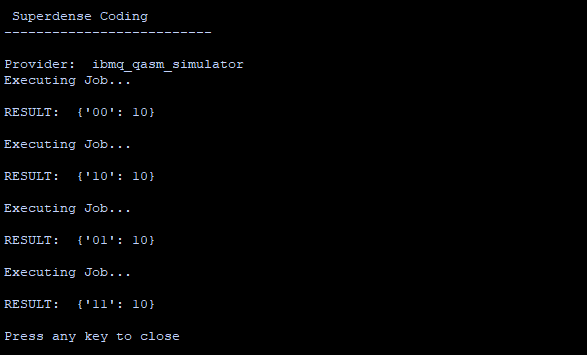Want to learn about Quantum Programming? Head over to Quantum Computing UK: https://quantumcomputinguk.org/The Future of Materials Discovery: Reducing R&D Costs significantly with GenMat’s AI and Machine Learning Tools

When: July 13, 2023 at 11:30am

What: GenMat Webinar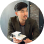#### Jake Vikoren

Company Speaker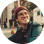Company Speaker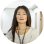#### Araceli Venegas

Company Speaker## Relevant

### Cambridge Quantum Computing Announces Integration of tket Platform Into Strangeworks Ecosystem## RecommendedThe Future of Materials Discovery: Reducing R&D Costs significantly with GenMat’s AI and Machine Learning Tools

When: July 13, 2023 at 11:30am

What: GenMat Webinar#### Jake Vikoren

Company SpeakerCompany Speaker#### Araceli Venegas

Company SpeakerKeep track of everything going on in the Quantum Technology Market.

In one place.

## Related Articles## July 28, 2021## January 5, 2021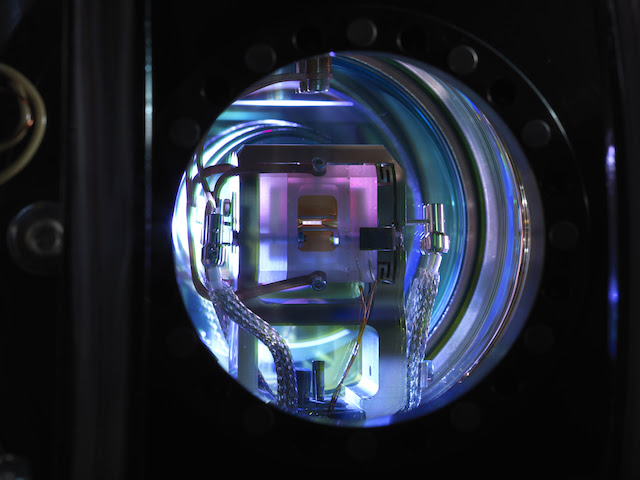## December 8, 2023## December 8, 2023## December 6, 2023## December 7, 2023

Explore our intelligence solutions